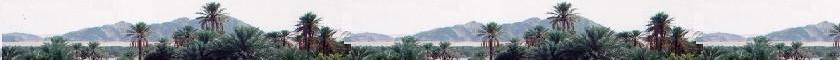Quantum Mechanics

Schrodinger equation

Quantum Mechanics

Propagators : Pg

Quantum Simple Harmonic
Oscillator QSHO

Quantum Mechanics
Simulation With GNU Octave

© The scientific sentence. 2010

# Many-electron atoms: Non-interacting particles

### 1. The multi-particle system :

The Hamiltonian of an N-particle system is:
H(r1, r2, ..., rN,t) = Σ(i) Pi2/2mi + V(r1, r2, ..., rN,t)
i from 1 to N

The term :
Σ(i) Pi2/2mi represents the total kinetic energy of the system.

The term:
V(r1, r2, ..., rN,t) represents the interaction between the particles making up the system, and the interaction of these particles with the outside of the system.

If the particles do not interact with one another, then V(r1, r2, ..., rN,t) will represent only the interaction of the particles with the outside of the system. (The outside of the system of N electrons can be the nucleus of the atom they constitute). Therefore, the common potential becomes:

V(r1, r2, ..., rN,t) = Σ(i) V(ri,t)
i from 1 to N

The total Hamiltonian becomes:

H(r1, r2, ..., rN,t) = Σ(i) Pi2/2mi + Σ(i) V(ri,t)
= Σ(i) {Pi2/2mi + V(ri,t)} = Σ(i) Hi(ri,t)
i from 1 to N

Where

Hi(ri,t) = Pi2/2mi + V(ri,t)

Therefore
For non-interacting particles, the N-particle Hamiltonian of the system is the sum of N independent single-particle Hamiltonians. The energies of the particles making up the system are independent; and their instantaneous positions are also not related. Hence, the N-particle system wave function ψ(r1, r2, ..., rN,t) is written as the product of N-independent single-particle wave-functions:

ψ(r1, r2, ..., rN,t) = ψ1(r1,t) ψ2(r2,t) ... ψN(rN,t) = Π(i) ψi(ri,t)
i from 1 to N

Let's recall |ψi(ri,t)|2 is the probability of finding the ith particle at the time t between ri and ri + dri. Since it is independent and completely unaffected by the others, this probability must satisfy the following normalization condition:

∫|ψi(ri,t)|2 dri = 1
dri from - ∞ to + ∞

The probability of finding the whole N-independent particle system at the time t with each ith particle between its ri and ri + dri is |ψ(r1, r2, ..., rN,t)|2; that must satisfy the following normalization condition:

∫|ψ(r1, r2, ..., rN,t))|2 dr1 dr2 ... drN = 1
dri from - ∞ to + ∞

The time-dependent Schrodinger equation for this whole system of N non-interacting particles is:

iħ ∂ ψ(r1, r2, ..., rN,t)/∂t = H ψ(r1, r2, ..., rN,t)

The corresponding multi-particle state of definite energy E is:

ψ(r1, r2, ..., rN,t) = ψE(r1, r2, ..., rN) exp{- iEt/ħ}

ψ(r1, r2, ..., rN,t) is the eigenstate of the Hamiltonian H of the system.
ψE(r1, r2, ..., rN) is the stationary state of the Hamiltonian H of this system.
E is the eigenvalue of the Hamiltonian H of the system.

The stationary wave-function ψE(r1, r2, ..., rN) of the system satisfies the time-independent Schrodinger equation

E(r1, r2, ..., rN) = E ψE(r1, r2, ..., rN)

The time-dependent Schrodinger equation for this system of N non-interacting particles is then written as a set of N independent equations, for each particle, of the form :

iħ ∂ψi(ri,t)/∂ = Hi ψi(ri,t)
i from 1 to N

In the case of the potential is time-independent, we have:
V(ri,t) = V(ri)

ψ(ri,t) = ψE(ri) exp{- iEit/ħ}

ψ(ri,t) is the eigenstate of the Hamiltonian Hi of one particle.
ψE(ri) is the stationary state of the Hamiltonian Hi of this particle.
Ei is the eigenvalue of the Hamiltonian Hi of this particle.

The stationary wave-function ψE(ri) of the particle satisfies the time-independent Schrodinger equation:

HiψEi(ri) = Ei ψEi(ri)

We have finally:
ψE(r1, r2, ..., rN) = Π(i) ψEi(ri) =
ψE1(r1) ψE2(r2) ... ψEN(rN)
i from 1 to N

and
E = Σ(i) Ei = E1 + E2 + ... + EN
i from 1 to N

ψE(r1, r2, ..., rN) = ψE1(r1) ψE2(r2) ... ψEN(rN)

E = Σ(i) Ei = E1 + E2 + ... + EN

i from 1 to N

For non identical and non-interacting particles, the wave function of the whole system is the product of the wave functions of the component particles; and the energy of the whole system is the sum of the energies of the component particles.

 chimie labs | Physics and Measurements | Probability & Statistics | Combinatorics - Probability | Chimie | Optics | contact |